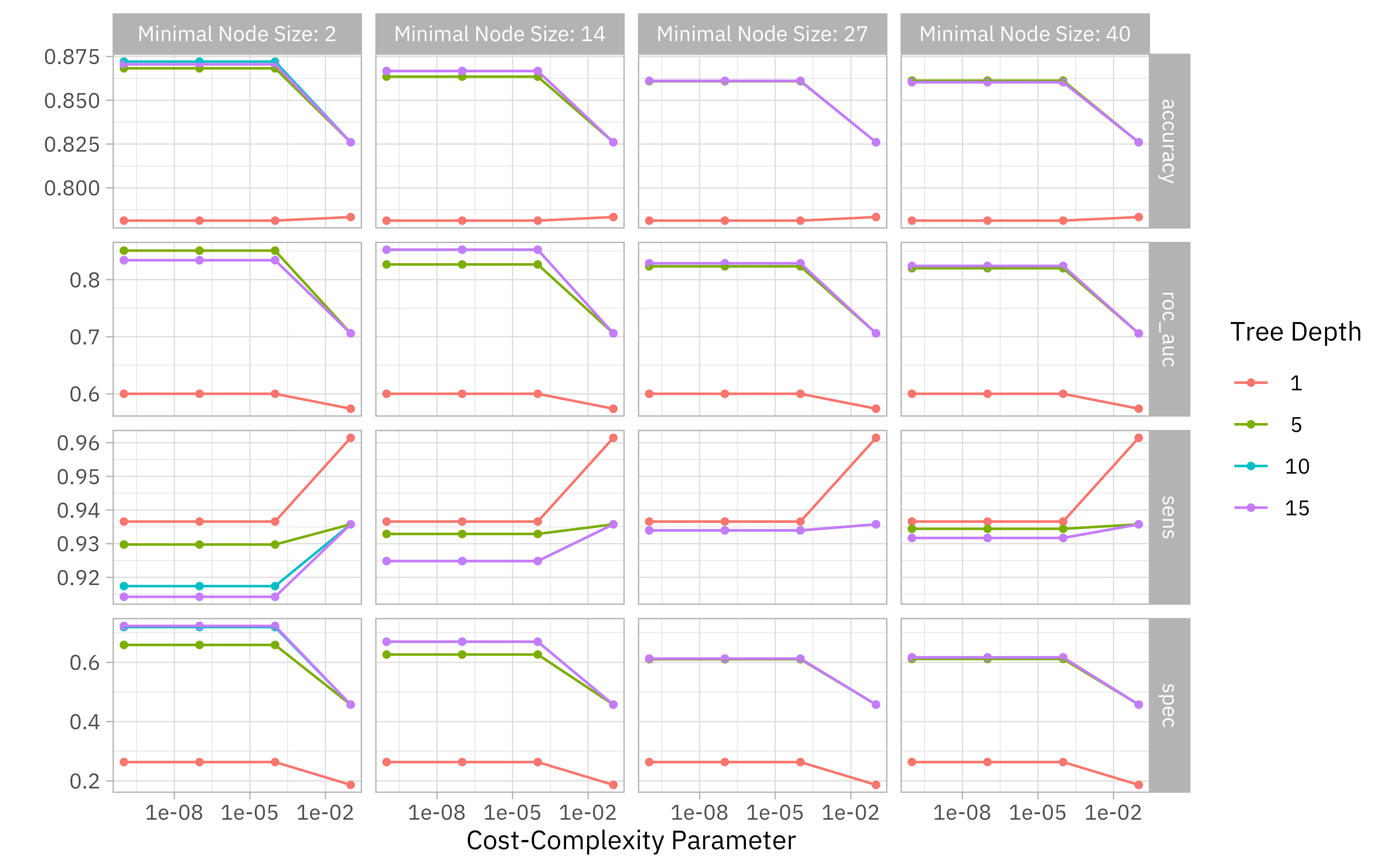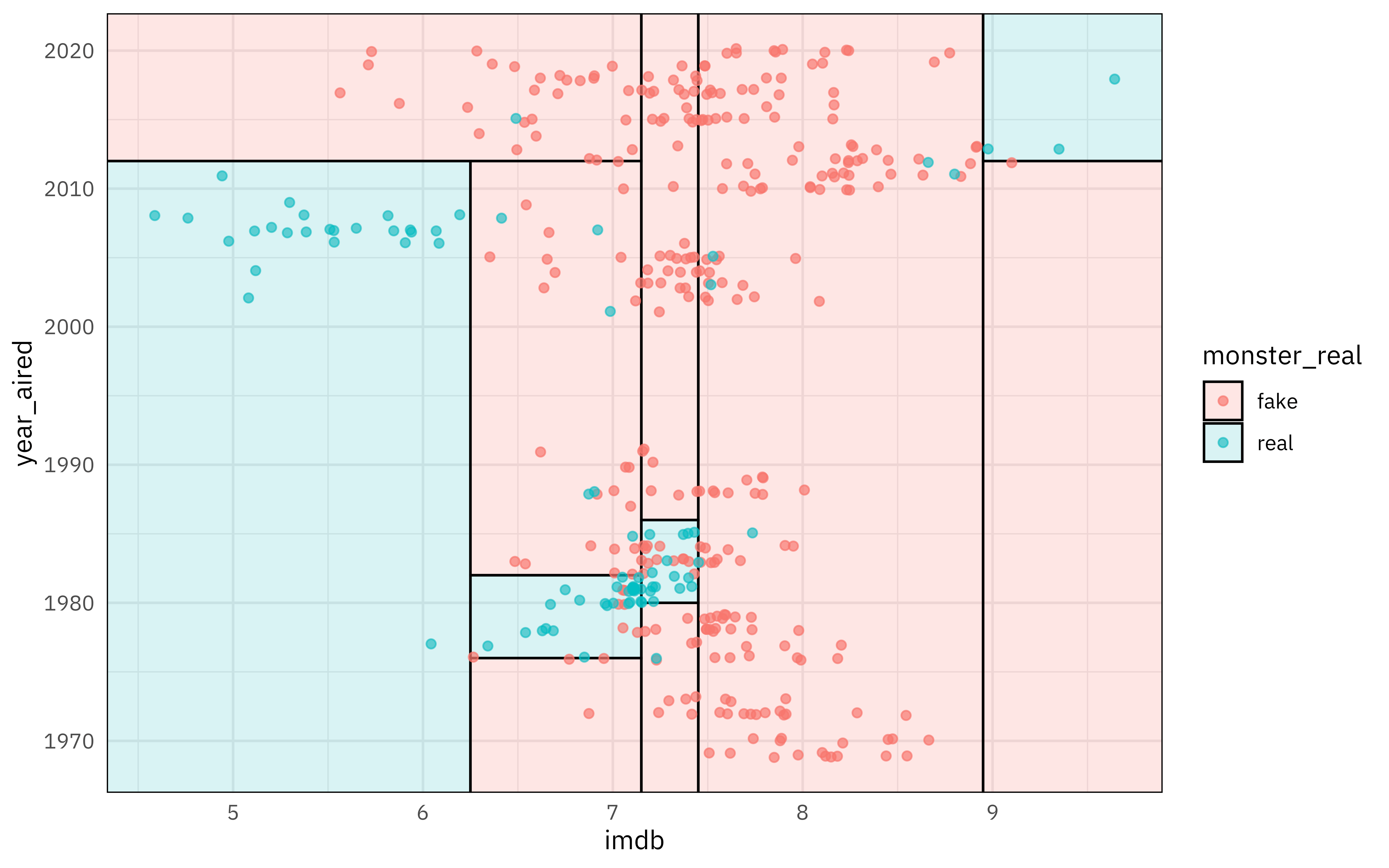Want to share your content on R-bloggers? click here if you have a blog, or here if you don't.

This is the latest in my series of screencasts demonstrating how to use the tidymodels packages, from just getting started to tuning more complex models. Today’s screencast walks through how to train and evalute a random forest model, with this week’s `#TidyTuesday` dataset on Scooby Doo episodes. ?

Here is the code I used in the video, for those who prefer reading instead of or in addition to video.

## Explore data

Our modeling goal is to predict which Scooby Doo monsters are real and which are not, based on other characteristics of the episode.

```library(tidyverse)

scooby_raw %>%
filter(monster_amount > 0) %>%
count(monster_real)

## # A tibble: 2 x 2
##   monster_real     n
##   <chr>        <int>
## 1 FALSE          404
## 2 TRUE           112
```

Most monsters are not real!

How did the number of real vs. fake monsters change over the decades?

```scooby_raw %>%
filter(monster_amount > 0) %>%
count(
year_aired = 10 * ((lubridate::year(date_aired) + 1) %/% 10),
monster_real
) %>%
mutate(year_aired = factor(year_aired)) %>%
ggplot(aes(year_aired, n, fill = monster_real)) +
geom_col(position = position_dodge(preserve = "single"), alpha = 0.8) +
labs(x = "Date aired", y = "Monsters per decade", fill = "Real monster?")
```How are these different episodes rated on IMDB?

```scooby_raw %>%
filter(monster_amount > 0) %>%
mutate(imdb = parse_number(imdb)) %>%
ggplot(aes(imdb, after_stat(density), fill = monster_real)) +
geom_histogram(position = "identity", alpha = 0.5) +
labs(x = "IMDB rating", y = "Density", fill = "Real monster?")
```It looks like there are some meaningful relationships there that we can use for modeling, but they are not linear so a decision tree may be a good fit.

## Build and tune a model

Let’s start our modeling by setting up our “data budget.” We’re only going to use the year each episode was aired and the episode rating.

```library(tidymodels)

set.seed(123)
scooby_split <- scooby_raw %>%
mutate(
imdb = parse_number(imdb),
year_aired = lubridate::year(date_aired)
) %>%
filter(monster_amount > 0, !is.na(imdb)) %>%
mutate(
monster_real = case_when(
monster_real == "FALSE" ~ "fake",
TRUE ~ "real"
),
monster_real = factor(monster_real)
) %>%
select(year_aired, imdb, monster_real, title) %>%
initial_split(strata = monster_real)
scooby_train <- training(scooby_split)
scooby_test <- testing(scooby_split)

set.seed(234)
scooby_folds <- bootstraps(scooby_train, strata = monster_real)
scooby_folds

## # Bootstrap sampling using stratification
## # A tibble: 25 x 2
##    splits            id
##    <list>            <chr>
##  1 <split [375/133]> Bootstrap01
##  2 <split [375/144]> Bootstrap02
##  3 <split [375/140]> Bootstrap03
##  4 <split [375/132]> Bootstrap04
##  5 <split [375/139]> Bootstrap05
##  6 <split [375/134]> Bootstrap06
##  7 <split [375/146]> Bootstrap07
##  8 <split [375/132]> Bootstrap08
##  9 <split [375/143]> Bootstrap09
## 10 <split [375/143]> Bootstrap10
## # … with 15 more rows
```

Next, let’s create our decision tree specification. It is tunable, and we could not fit this right away to data because we haven’t said what the model parameters are yet.

```tree_spec <-
decision_tree(
cost_complexity = tune(),
tree_depth = tune(),
min_n = tune()
) %>%
set_mode("classification") %>%
set_engine("rpart")

tree_spec

## Decision Tree Model Specification (classification)
##
## Main Arguments:
##   cost_complexity = tune()
##   tree_depth = tune()
##   min_n = tune()
##
## Computational engine: rpart
```

Let’s set up a grid of possible model parameters to try.

```tree_grid <- grid_regular(cost_complexity(), tree_depth(), min_n(), levels = 4)
tree_grid

## # A tibble: 64 x 3
##    cost_complexity tree_depth min_n
##              <dbl>      <int> <int>
##  1    0.0000000001          1     2
##  2    0.0000001             1     2
##  3    0.0001                1     2
##  4    0.1                   1     2
##  5    0.0000000001          5     2
##  6    0.0000001             5     2
##  7    0.0001                5     2
##  8    0.1                   5     2
##  9    0.0000000001         10     2
## 10    0.0000001            10     2
## # … with 54 more rows
```

Now let’s fit each possible parameter combination to each resample. By putting non-default metrics into `metric_set()`, we can specify which metrics are computed for each resample.

```doParallel::registerDoParallel()

set.seed(345)
tree_rs <-
tune_grid(
tree_spec,
monster_real ~ year_aired + imdb,
resamples = scooby_folds,
grid = tree_grid,
metrics = metric_set(accuracy, roc_auc, sensitivity, specificity)
)

tree_rs

## # Tuning results
## # Bootstrap sampling using stratification
## # A tibble: 25 x 4
##    splits            id          .metrics           .notes
##    <list>            <chr>       <list>             <list>
##  1 <split [375/133]> Bootstrap01 <tibble [256 × 7]> <tibble [0 × 1]>
##  2 <split [375/144]> Bootstrap02 <tibble [256 × 7]> <tibble [0 × 1]>
##  3 <split [375/140]> Bootstrap03 <tibble [256 × 7]> <tibble [0 × 1]>
##  4 <split [375/132]> Bootstrap04 <tibble [256 × 7]> <tibble [0 × 1]>
##  5 <split [375/139]> Bootstrap05 <tibble [256 × 7]> <tibble [0 × 1]>
##  6 <split [375/134]> Bootstrap06 <tibble [256 × 7]> <tibble [0 × 1]>
##  7 <split [375/146]> Bootstrap07 <tibble [256 × 7]> <tibble [0 × 1]>
##  8 <split [375/132]> Bootstrap08 <tibble [256 × 7]> <tibble [0 × 1]>
##  9 <split [375/143]> Bootstrap09 <tibble [256 × 7]> <tibble [0 × 1]>
## 10 <split [375/143]> Bootstrap10 <tibble [256 × 7]> <tibble [0 × 1]>
## # … with 15 more rows
```

All done!

## Evaluate and understand our model

Now that we have tuned our decision tree model, we can choose which set of model parameters we want to use. What are some of the best options?

```show_best(tree_rs)

## # A tibble: 5 x 9
##   cost_complexity tree_depth min_n .metric  .estimator  mean     n std_err
##             <dbl>      <int> <int> <chr>    <chr>      <dbl> <int>   <dbl>
## 1    0.0000000001         10     2 accuracy binary     0.872    25 0.00481
## 2    0.0000001            10     2 accuracy binary     0.872    25 0.00481
## 3    0.0001               10     2 accuracy binary     0.872    25 0.00481
## 4    0.0000000001         15     2 accuracy binary     0.871    25 0.00456
## 5    0.0000001            15     2 accuracy binary     0.871    25 0.00456
## # … with 1 more variable: .config <chr>
```

We can visualize all of the combinations we tried.

```autoplot(tree_rs) + theme_light(base_family = "IBMPlexSans")
```If we used `select_best()`, we would pick the numerically best option. However, we might want to choose a different option that is within some criteria of the best performance, like a simpler model that is within one standard error of the optimal results. We finalize our model just like we finalize a workflow, as shown in previous posts.

```simpler_tree <- select_by_one_std_err(tree_rs,
-cost_complexity,
metric = "roc_auc"
)

final_tree <- finalize_model(tree_spec, simpler_tree)
```

Now we can fit `final_tree` to our training data.

```final_fit <- fit(final_tree, monster_real ~ year_aired + imdb, scooby_train)
```

We also could use `last_fit()` instead of `fit()`, by swapping out the split for the training data. This will fit one time on the training data and evaluate one time on the testing data.

```final_rs <- last_fit(final_tree, monster_real ~ year_aired + imdb, scooby_split)
```

This is the first time we have used the testing data through this whole analysis, and let’s us see how our model performs on the testing data. A bit worse, unfortunately!

```collect_metrics(final_rs)

## # A tibble: 2 x 4
##   .metric  .estimator .estimate .config
##   <chr>    <chr>          <dbl> <chr>
## 1 accuracy binary         0.857 Preprocessor1_Model1
## 2 roc_auc  binary         0.780 Preprocessor1_Model1
```

Finally, we can use the parttree package to visualize our decision tree results.

```library(parttree)

scooby_train %>%
ggplot(aes(imdb, year_aired)) +
geom_parttree(data = final_fit, aes(fill = monster_real), alpha = 0.2) +
geom_jitter(alpha = 0.7, width = 0.05, height = 0.2, aes(color = monster_real))
```